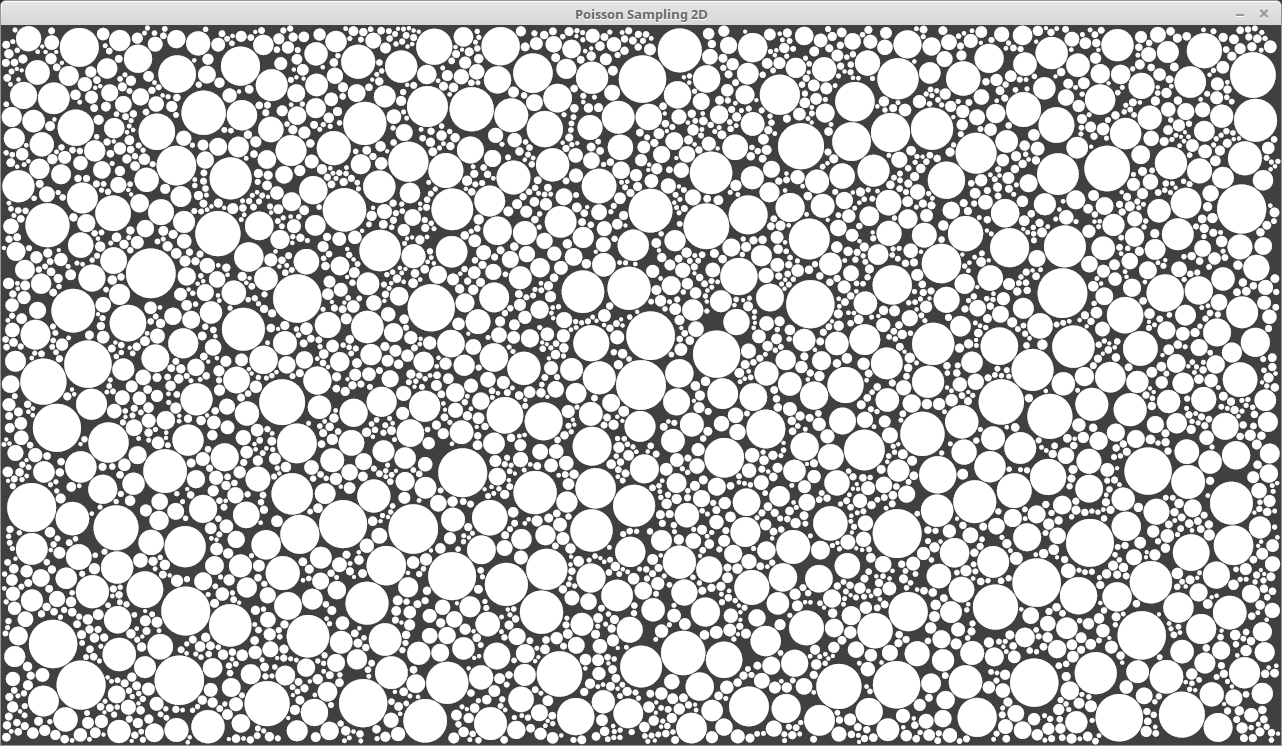Thomas Diewald is a prolific graphics programmer in Java, Javascript, OpenGL/WebGL, GLSL, OpenCL, C++, C. He has developed a number of libraries for vanilla processing, and we explore their use in JRubyArt and propane (actually we barely scratch the surface) in this and in recent previous posts, but hope that we can provide inspiration for others to follow.

### PixelFlow library

A Processing/Java library for high performance GPU-Computing (GLSL). See PixelFlow library on github and the PixelFlow website.

Here an example sketch translated for JRubyArt for the sketch to run you need install the `PixelFlow` libraries using the processing ide, I suppose there could be a possibility of a gem like toxiclibs. This example demonstrates how to create an anonymous class instance in JRuby that implements an abstract java class:-

``````#
# PixelFlow | Copyright (C) 2016 Thomas Diewald - http://thomasdiewald.com
# Translated to RubyArt by Martin Prout
# A Processing/Java library for high performance GPU-Computing (GLSL).
#

java_import 'com.thomasdiewald.pixelflow.java.sampling.PoissonSample'
java_import 'com.thomasdiewald.pixelflow.java.sampling.PoissonDiscSamping2D'

def settings
size(1280, 720, P2D)
smooth(16)
end

def setup
sketch_title 'Poisson Sampling 2D'
generate_poisson_sampling2d
end

def generate_poisson_sampling2d
# create an anonymous class instance in JRuby that implements the java
# abstract class PoissonDiscSamping2D
pds = Class.new(PoissonDiscSamping2D) do
def newInstance(x, y, r, rcollision)
PoissonSample.new(x, y, r, rcollision)
end
end.new
bounds = [0, 0, 0, width, height, 0]
rmin = 2
rmax = 25
roff = 0.5
new_points = 100
start = Time.now
pds.setRandomSeed(rand(0..10_0000))
pds.generatePoissonSampling2D(bounds, rmin, rmax, roff, new_points)
@samples = pds.samples
time = Time.now - start
puts("poisson samples 2D generated")
puts("    time: #{(time * 1000).floor}ms")
puts("    count: #{samples.size}")
end

def draw
background(64)
samples.each do |sample|
px = sample.x
py = sample.y
stroke(255)
stroke_weight(0.5)
fill(255)
no_stroke
ellipse(px, py, pr * 2, pr * 2)
else
stroke(255)
stroke_weight(2)
point(px, py)
end
end
end

def key_released
case key
when ' '